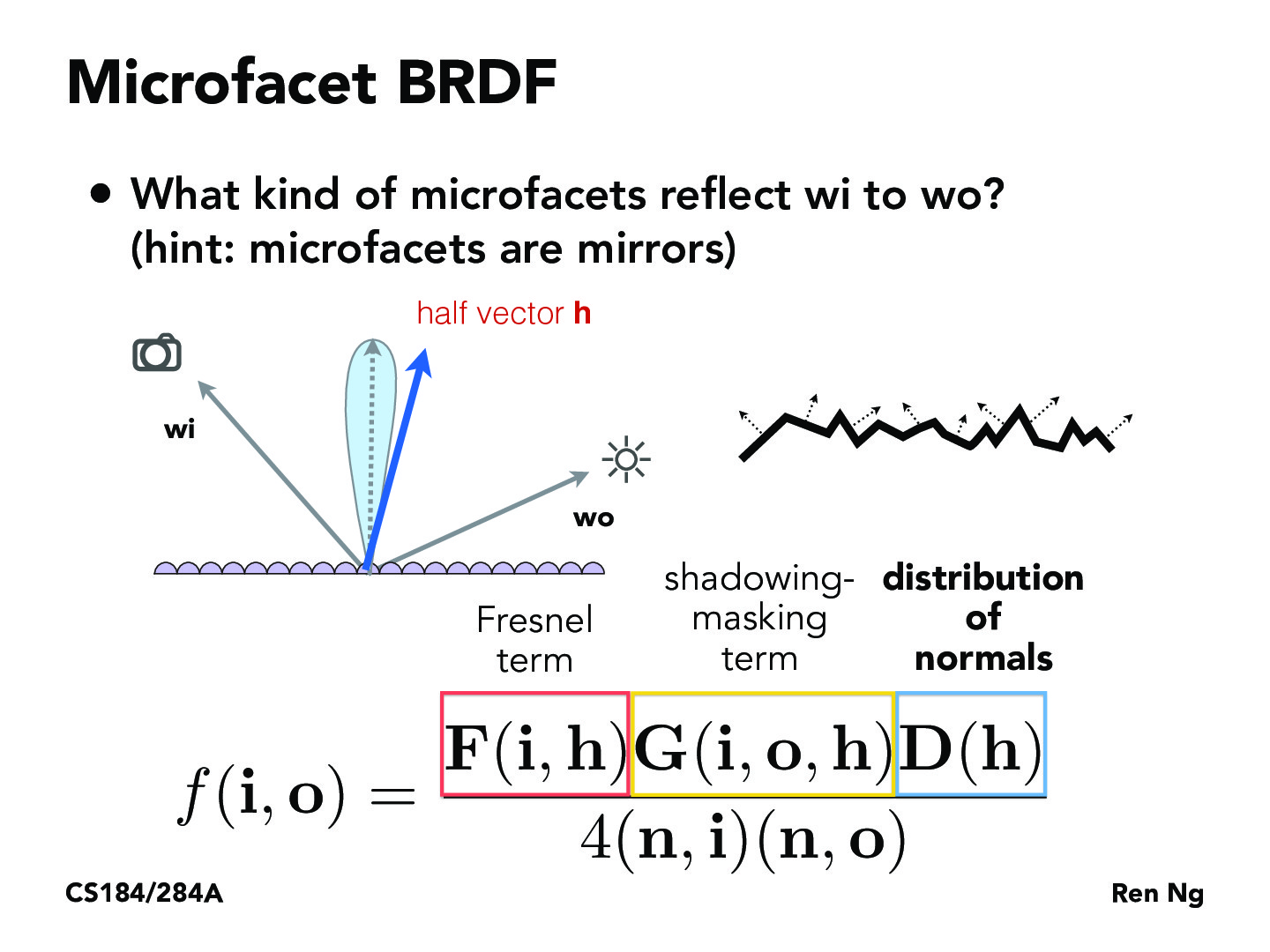Lecture 14: Intro to Material Modeling (26)Huw-man

what is the 4(n,i)(n,o) term in the denominator?katamarisun

Great question! Surprisingly there's actually quite a bit of diversity in the actual terms involved in the bottom, but here's a brief intro to the terms used in this slide:

Rense, W. A. (1950). doi:10.1364/josa.40.000055 Explains the 4(N*O) term, which is really derived from the fact that when sampling f(I,o), the path tracer is sampling with respect to the input angle wi; but when actually integrating over the BRDF, we're integrating with respect to the solid angles wh, or the half vectors. Figure 3 gives a good illustration of how these two differentials, dwi and dwh are related.

http://cs.uns.edu.ar/cg/clasespdf/p192-blinn.pdf With respect to (N*I), there's the idea that when there's a tilt between the surface and our eye / the outgoing direction wo, the observer will be exposed to a greater surface area of the actual micro facets properly oriented for specular reflection, hence the term.

As far as consistency, http://inst.cs.berkeley.edu/~cs294-13/fa09/lectures/cookpaper.pdf this paper actually suggests using pi instead of 4. And you'll notice that both the first two papers do not actually include the effect of the other! Thus it's important to note that some of these terms were chosen heuristically rather than on any physical, or purely theoretical foundation. Hope you find these readings interesting!jamesyc23

Would the shadowing-masking term depend on the distribution of normals? It seems like (intuitively) a surface with a large variance in the distribution of surface normals would have a greater shadowing-masking term (the two don't seem independent).

You must be enrolled in the course to comment